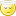[This article was first published on Keep on Fighting! » R Language, and kindly contributed to R-bloggers]. (You can report issue about the content on this page here)
Want to share your content on R-bloggers? click here if you have a blog, or here if you don't.

Yanping Chen raised a question in the Chinese COS forum on the output of Eviews: how to (re)format the decimal coefficients in equations as text output? For example, we want to round the numbers in `CC = 16.5547557654 + 0.0173022117998*PP + 0.216234040485 * PP(-1) + 0.810182697599 * (WP + WG)` to the 3rd decimal places. This can be simply done by regular expressions, as decimals always begin with a “`.`”. The basic steps are:

1. find out where are the decimals in the character string;
2. format them;
3. replace the original decimals with formatted values;

Given a character vector, we can format the decimals with the code below:

```# x: equations; FUN: formatting function; ...: passed to FUN
coefFormat = function(x, FUN, ...) {
sapply(x, function(s) {
dig = sapply(gregexpr("\.[0-9]+", s), function(m) {
sapply(seq(along = m), function(i) {
substr(s, m[i], m[i] + attr(m, "match.length")[i] - 1)
})
})
for (j in {
if (is.null(dim(dig)))
NULL
else 1:dim(dig)
}) {
s = sub(dig[j, 1], substring(FUN(as.numeric(dig[j, 1]), ...),
2), s, fixed = TRUE)
}
s
})
}```

I used `sapply()` for 3 times to avoid explicit loops but consequently the code might be difficult to read. The critical part is the regular expression “`\.[0-9]+`” which means one of more (controlled by “`+`” after “`[0-9]`”) digits (“`[0-9]`” or “`[:digit:]`”) after a decimal point “`.`”. As “`.`” is a metacharacter in regular expressions, we need to use a backslash before it, and again, “” is a special character in R, so we need another backslash to denote a backslash.```x = readLines(zz <- textConnection(
"CC = 16.5547557654 + 0.0173022117998 * PP + 0.216234040485 * PP(-1) + 0.810182697599 * (WP + WG)

II = 20.2782089394 + 0.150221823899 * PP + 0.61594357734 * PP(-1) - 0.157787636546 * KK

WP = 1.50029688603 + C(10) * XX + 0.146673821502 * XX(-1) + 0.130395687204 * AA
"))
close(zz)

writeLines(coefFormat(x, round, digits = 3))
#  CC = 16.555 + 0.017 * PP + 0.216 * PP(-1) + 0.81 * (WP + WG)
#
#  II = 20.278 + 0.15 * PP + 0.616 * PP(-1) - 0.158 * KK
#
#  WP = 1.5 + C(10) * XX + 0.147 * XX(-1) + 0.13 * AA
#
writeLines(coefFormat(x, formatC, digits = 3, format = "f"))
#  CC = 16.555 + 0.017 * PP + 0.216 * PP(-1) + 0.810 * (WP + WG)
#
#  II = 20.278 + 0.150 * PP + 0.616 * PP(-1) - 0.158 * KK
#
#  WP = 1.500 + C(10) * XX + 0.147 * XX(-1) + 0.130 * AA
#```